Question

# An ideal monatomic gas goes from P1 = 150 atm and V1 = 25 m3 to...

An ideal monatomic gas goes from P1 = 150 atm and V1 = 25 m3 to P2 and V2 via an adiabatic process. If P2 = 40 atm, what is V2 in m3?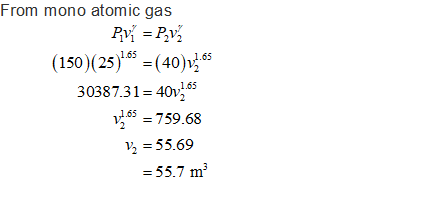#### Earn Coins

Coins can be redeemed for fabulous gifts.

Similar Homework Help Questions
• ### An ideal monatomic gas goes from P1 = 140 atm and V1 = 55 m3 to...

An ideal monatomic gas goes from P1 = 140 atm and V1 = 55 m3 to P2 and V2 via an adiabatic process. If P2 = 60 atm, what is V2 in m3?

• ### A gas is compressed from V1 = 0.3 m3, p1 = 1 bar to V2 =...

A gas is compressed from V1 = 0.3 m3, p1 = 1 bar to V2 = 0.1 m3, p2 = 1.9 bar. Pressure and volume are related linearly during the process. For the gas, find the work, in kJ.

• ### If (P1 = 3 atm, V1 = 0.2 m3) and (P4 = 1 atm, V4 =...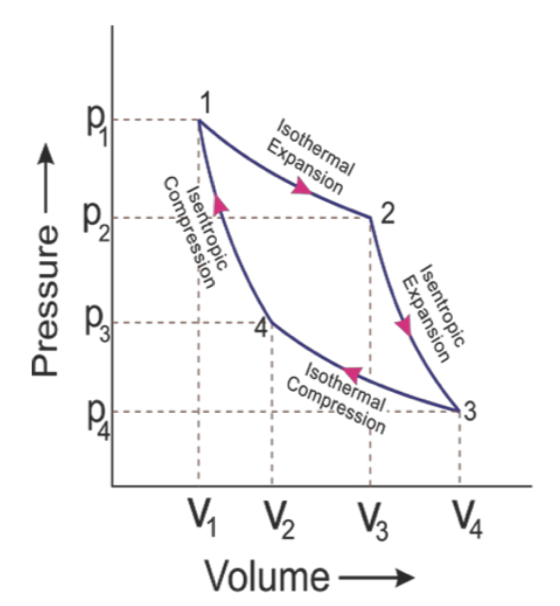If (P1 = 3 atm, V1 = 0.2 m3) and (P4 = 1 atm, V4 = 0.4 m3) and the heat absorbed by 12 mol of and ideal gas undergoing the Carnot cycle is 10 kJ, what is the work done? AIsothe Expansion 2 Isothermal Compression Volume →

• ### A sample of gas expands from V1 = 1.1 m3 and p1 = 46 Pa to...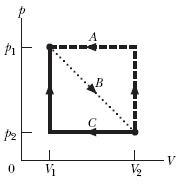A sample of gas expands from V1 = 1.1 m3 and p1 = 46 Pa to V2 = 4.8 m3 and p2 = 18 Pa along path B in the p-V diagram in the figure below. It is then compressed back to V1 along either path A or path C. Compute the net work done by the gas for the complete cycle along (a) path BA and (b) path BC. 0

• ### ss.19.2.8 The picture shows a circular process of an ideal monatomic gas in the amount of...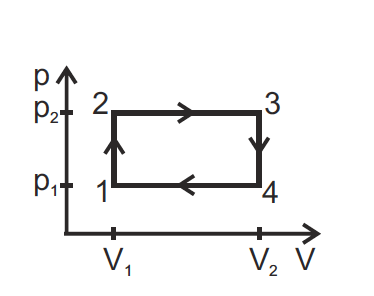ss.19.2.8 The picture shows a circular process of an ideal monatomic gas in the amount of 1 mole in the coordinates (p,V). In which sections of the process does the gas absorb heat and in which sections does it release heat? Find the efficiency of the cycle. The pressures p1, p2 and volumes V1, V2 are given. a clear explanation is highly appreciated! Thanks! vd

• ### If the engine uses 5 moles of an ideal gas what are the volumes: V1, V2,...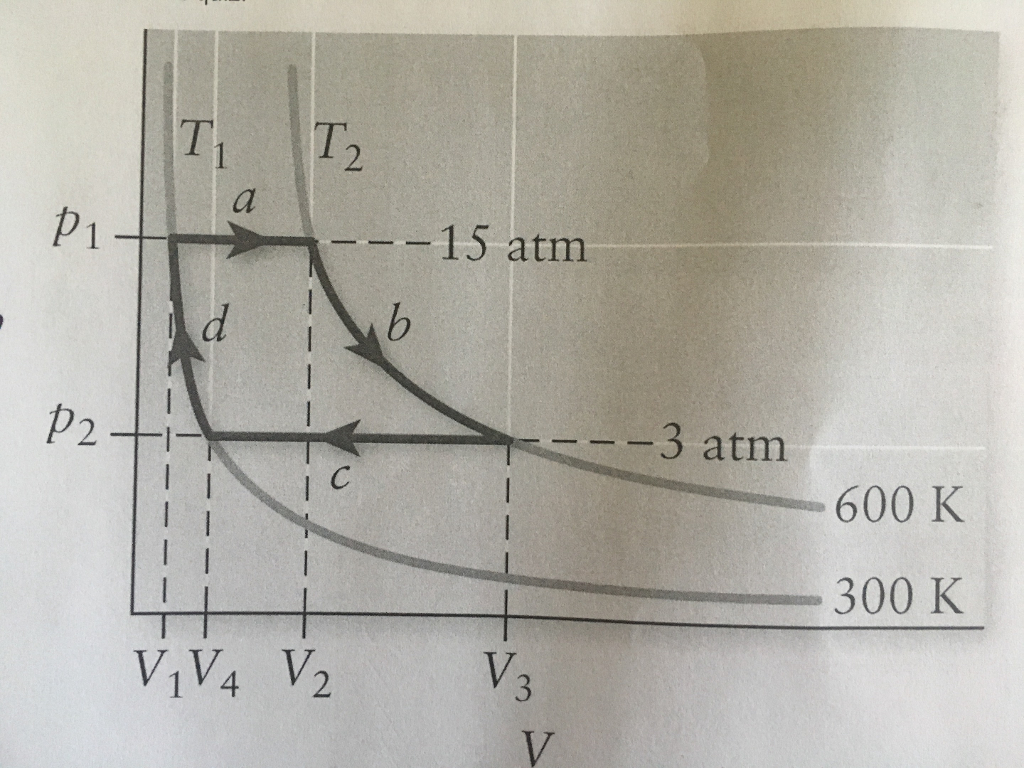If the engine uses 5 moles of an ideal gas what are the volumes: V1, V2, V3, and V4? T, IT, p1 15 atm p2 3 atm 600 K 300 K 1V4 V2

• ### A sample (8.1 mol) of an ideal monatomic gas goes through the process shown in Figure...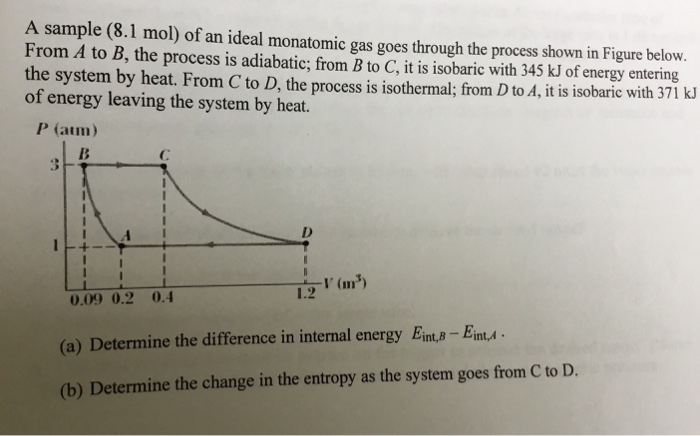A sample (8.1 mol) of an ideal monatomic gas goes through the process shown in Figure below. From A to B, the process is adiabatic; from B to C, it is isobaric with 345 kJ of energy entering the system by heat. From C to D,the process is isothermal from D to d,itis sobarie with 3711 of energy leaving the system by heat. P (atm) 12 (m') 0.09 0.2 0.4 (a) Determine the difference in internal energy Eint,B-EinA (b) Determine...

• ### Dmole of an ideal gas follows the cycle shown in the figure. 1-2 is isochoric process,...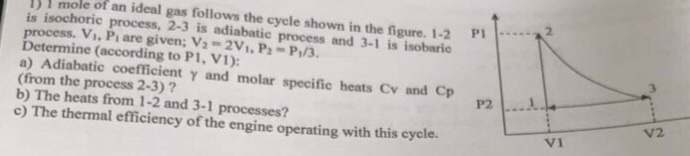Dmole of an ideal gas follows the cycle shown in the figure. 1-2 is isochoric process, 2-3 is adiabatic process and 3-1 is isobaric process. Vi, Pi are given; V2-2V, P2- P/3. Determine (according to P1, V1) a) Adiabatic coefficient y and molar specific heats Cv and Cp (from the process 2-3) ? b) The heats from 1-2 and 3-1 processes? c) The thermal efficiency of the engine operating with this cycle. PI P2 V2 VI .. Dmole of an...

• ### A 1.00-mol sample of an ideal monatomic gas, initially at a pressure of 1.00 atm and...

A 1.00-mol sample of an ideal monatomic gas, initially at a pressure of 1.00 atm and a volume of 0.025 0 m3 , is heated to a final state with a pressure of 2.00 atm. and a volume of 0.040 0 m3 . Determine the change in entropy of the gas in this process.

• ### 3.1 moles of ideal gas undergo an expansion from V1 = 1.2 m3 to V2 =...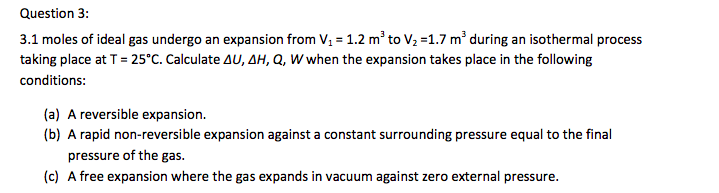3.1 moles of ideal gas undergo an expansion from V1 = 1.2 m3 to V2 = 1.7 m3 during an isothermal process taking place at T = 25 degree C. Calculate Delta U, Delta H, Q, W when The expansion takes place in The following conditions: A reversible expansion. A rapid non-reversible expansion against a constant surrounding pressure equal to The final pressure of The gas. A free expansion where The gas expands in vacuum against zero external pressure.